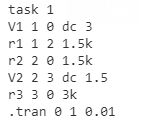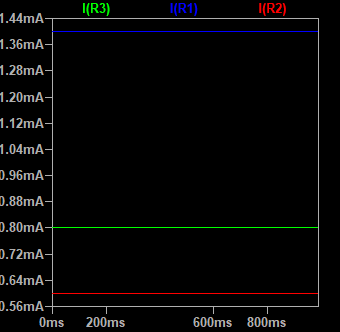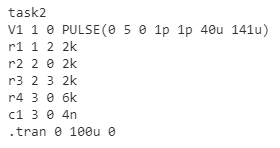ENGR338 Lab 2022 Fall
Lab 1 Review of Superposition, Thevenin's Equivalent Circuit, and LTSpice
Name:

Review of Superposition, Thevenin's Equivalent Circuit, and LTSpice

Introduction: This lab serves mainly to review circuit 1 material including resistance and voltage calculation as well as simplifying circuits using Thevenin's Equivalent Circuit theorum. Everything was checked using LTSpice
specifically using SPICE code which allows us to program the circuit into LTSpice without the need for symbols making it slightly more universal than using the symbols in LTSpice.

Materials: LTSpice

Methods: First, the superposition theory was used to seperate the first circuit into two individual circuits where the voltage drops could be calculated. These voltages were then added together to get the corresponding component values at each point in the circuit, the work can be seen below in Figure 1 of results. Then SPICE code was created for the circuit and the voltages were monitored at each node which allowed me to verify my answers.

For task 2, Thevenin's equivalent circuit thereom was used. Step one of this process is to remove the load, CL. The circuit was then simplified down to a single resistor and voltage source. This was done by first calculating R by finding the total overall resistance of the circuit. Next, V was calculated using our new R value times Vin. This can be seen below in Figure 3. Again, SPICE code was used to verify results.

Results:Figure 1. Hand calculations for task1Figure 2. SPICE Code for task 1Figure 3. LTSpice simulation result, Voltages at each node (Green - 1, Blue - 2, Red - 3)Fiugre 4.
LTSpice simulation result, Current at each node (Blue -1, Red -2 , Green - 3)Fiugre 5.